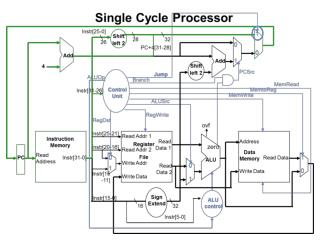DownloadDownload Presentation(a) add Rd, Rs, Rt Reg[Rd]=Reg[Rs]+Reg[Rt] (b) lw Rt, offs(Rs) Reg[Rt]=MEM[Reg[Rs]+offs]

# (a) add Rd, Rs, Rt Reg[Rd]=Reg[Rs]+Reg[Rt] (b) lw Rt, offs(Rs) Reg[Rt]=MEM[Reg[Rs]+offs]

Download Presentation## (a) add Rd, Rs, Rt Reg[Rd]=Reg[Rs]+Reg[Rt] (b) lw Rt, offs(Rs) Reg[Rt]=MEM[Reg[Rs]+offs]

- - - - - - - - - - - - - - - - - - - - - - - - - - - E N D - - - - - - - - - - - - - - - - - - - - - - - - - - -
##### Presentation Transcript

1. (a) add Rd, Rs, Rt Reg[Rd]=Reg[Rs]+Reg[Rt] (b) lw Rt, offs(Rs) Reg[Rt]=MEM[Reg[Rs]+offs] Instruction (a), all blocks except data memory are used for this instruction Instruction (b), all blocks are used for this instruction

2. Setting of the control signals

3. I-Mem, Add, Mux, ALU, Regs, D-Mem, Control 400ps, 100ps, 30ps, 120ps, 200ps, 350ps, 100ps 500ps, 150ps, 100ps, 180ps, 220ps, 1000ps, 65ps MIPS AND instruction: I-Mem + Regs + Mux + ALU +Mux + Regs = 400 + 200 + 30+ 120+30+ 200 = 980 ps MIPs LD instruction: I-Mem + Regs + Mux +ALU + D-Mem + Mux + Regs = 400+200+30+120+350+30+200 = 1330 ps MIPS BEQ instruction: I-Mem + Regs + Mux + ALU + Mux = 400 + 200 + 30 + 120 + 30 = 780 ps